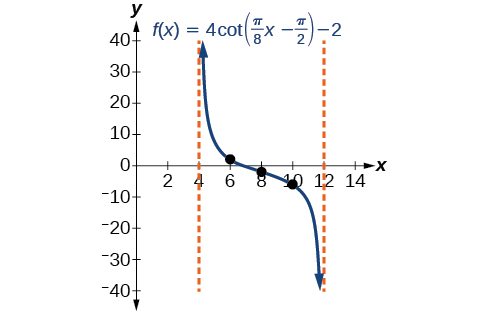# 8.2 Graphs of the other trigonometric functions  (Page 7/9)

 Page 7 / 9

The cotangent graph has vertical asymptotes at each value of $\text{\hspace{0.17em}}x\text{\hspace{0.17em}}$ where $\text{\hspace{0.17em}}\mathrm{tan}\text{\hspace{0.17em}}x=0;\text{\hspace{0.17em}}$ we show these in the graph below with dashed lines. Since the cotangent is the reciprocal of the tangent, $\text{\hspace{0.17em}}\mathrm{cot}\text{\hspace{0.17em}}x\text{\hspace{0.17em}}$ has vertical asymptotes at all values of $\text{\hspace{0.17em}}x\text{\hspace{0.17em}}$ where $\text{\hspace{0.17em}}\mathrm{tan}\text{\hspace{0.17em}}x=0,\text{\hspace{0.17em}}$ and $\text{\hspace{0.17em}}\mathrm{cot}\text{\hspace{0.17em}}x=0\text{\hspace{0.17em}}$ at all values of $\text{\hspace{0.17em}}x\text{\hspace{0.17em}}$ where $\text{\hspace{0.17em}}\mathrm{tan}\text{\hspace{0.17em}}x\text{\hspace{0.17em}}$ has its vertical asymptotes.

## Features of the graph of y = A Cot( Bx )

• The stretching factor is $\text{\hspace{0.17em}}|A|.$
• The period is $\text{\hspace{0.17em}}P=\frac{\pi }{|B|}.$
• The domain is $\text{\hspace{0.17em}}x\ne \frac{\pi }{|B|}k,\text{\hspace{0.17em}}$ where $\text{\hspace{0.17em}}k\text{\hspace{0.17em}}$ is an integer.
• The range is $\text{\hspace{0.17em}}\left(-\infty ,\infty \right).$
• The asymptotes occur at $\text{\hspace{0.17em}}x=\frac{\pi }{|B|}k,\text{\hspace{0.17em}}$ where $\text{\hspace{0.17em}}k\text{\hspace{0.17em}}$ is an integer.
• $y=A\mathrm{cot}\left(Bx\right)\text{\hspace{0.17em}}$ is an odd function.

## Graphing variations of y = cot x

We can transform the graph of the cotangent in much the same way as we did for the tangent. The equation becomes the following.

$y=A\mathrm{cot}\left(Bx-C\right)+D$

## Properties of the graph of y = A Cot( Bx −c)+ D

• The stretching factor is $\text{\hspace{0.17em}}|A|.$
• The period is $\text{\hspace{0.17em}}\frac{\pi }{|B|}.$
• The domain is $\text{\hspace{0.17em}}x\ne \frac{C}{B}+\frac{\pi }{|B|}k,$ where $\text{\hspace{0.17em}}k\text{\hspace{0.17em}}$ is an integer.
• The range is $\text{\hspace{0.17em}}\left(\mathrm{-\infty },\infty \right).$
• The vertical asymptotes occur at $\text{\hspace{0.17em}}x=\frac{C}{B}+\frac{\pi }{|B|}k,$ where $\text{\hspace{0.17em}}k\text{\hspace{0.17em}}$ is an integer.
• There is no amplitude.
• $y=A\mathrm{cot}\left(Bx\right)\text{\hspace{0.17em}}$ is an odd function because it is the quotient of even and odd functions (cosine and sine, respectively)

Given a modified cotangent function of the form $\text{\hspace{0.17em}}f\left(x\right)=A\mathrm{cot}\left(Bx\right),$ graph one period.

1. Express the function in the form $\text{\hspace{0.17em}}f\left(x\right)=A\mathrm{cot}\left(Bx\right).$
2. Identify the stretching factor, $\text{\hspace{0.17em}}|A|.$
3. Identify the period, $\text{\hspace{0.17em}}P=\frac{\pi }{|B|}.$
4. Draw the graph of $\text{\hspace{0.17em}}y=A\mathrm{tan}\left(Bx\right).$
5. Plot any two reference points.
6. Use the reciprocal relationship between tangent and cotangent to draw the graph of $\text{\hspace{0.17em}}y=A\mathrm{cot}\left(Bx\right).$
7. Sketch the asymptotes.

## Graphing variations of the cotangent function

Determine the stretching factor, period, and phase shift of $\text{\hspace{0.17em}}y=3\mathrm{cot}\left(4x\right),\text{\hspace{0.17em}}$ and then sketch a graph.

• Step 1. Expressing the function in the form $\text{\hspace{0.17em}}f\left(x\right)=A\mathrm{cot}\left(Bx\right)\text{\hspace{0.17em}}$ gives $\text{\hspace{0.17em}}f\left(x\right)=3\mathrm{cot}\left(4x\right).$
• Step 2. The stretching factor is $\text{\hspace{0.17em}}|A|=3.$
• Step 3. The period is $\text{\hspace{0.17em}}P=\frac{\pi }{4}.$
• Step 4. Sketch the graph of $\text{\hspace{0.17em}}y=3\mathrm{tan}\left(4x\right).$
• Step 5. Plot two reference points. Two such points are $\text{\hspace{0.17em}}\left(\frac{\pi }{16},3\right)\text{\hspace{0.17em}}$ and $\text{\hspace{0.17em}}\left(\frac{3\pi }{16},-3\right).$
• Step 6. Use the reciprocal relationship to draw $\text{\hspace{0.17em}}y=3\mathrm{cot}\left(4x\right).$
• Step 7. Sketch the asymptotes, $\text{\hspace{0.17em}}x=0,\text{\hspace{0.17em}}\text{\hspace{0.17em}}x=\frac{\pi }{4}.$

The orange graph in [link] shows $\text{\hspace{0.17em}}y=3\mathrm{tan}\left(4x\right)\text{\hspace{0.17em}}$ and the blue graph shows $\text{\hspace{0.17em}}y=3\mathrm{cot}\left(4x\right).$

Given a modified cotangent function of the form $\text{\hspace{0.17em}}f\left(x\right)=A\mathrm{cot}\left(Bx-C\right)+D,\text{\hspace{0.17em}}$ graph one period.

1. Express the function in the form $\text{\hspace{0.17em}}f\left(x\right)=A\mathrm{cot}\left(Bx-C\right)+D.$
2. Identify the stretching factor, $\text{\hspace{0.17em}}|A|.$
3. Identify the period, $\text{\hspace{0.17em}}P=\frac{\pi }{|B|}.$
4. Identify the phase shift, $\text{\hspace{0.17em}}\frac{C}{B}.$
5. Draw the graph of $\text{\hspace{0.17em}}y=A\mathrm{tan}\left(Bx\right)\text{\hspace{0.17em}}$ shifted to the right by $\text{\hspace{0.17em}}\frac{C}{B}\text{\hspace{0.17em}}$ and up by $\text{\hspace{0.17em}}D.$
6. Sketch the asymptotes $\text{\hspace{0.17em}}x=\frac{C}{B}+\frac{\pi }{|B|}k,$ where $\text{\hspace{0.17em}}k\text{\hspace{0.17em}}$ is an integer.
7. Plot any three reference points and draw the graph through these points.

## Graphing a modified cotangent

Sketch a graph of one period of the function $\text{\hspace{0.17em}}f\left(x\right)=4\mathrm{cot}\left(\frac{\pi }{8}x-\frac{\pi }{2}\right)-2.$

• Step 1. The function is already written in the general form $\text{\hspace{0.17em}}f\left(x\right)=A\mathrm{cot}\left(Bx-C\right)+D.$
• Step 2. $\text{\hspace{0.17em}}A=4,$ so the stretching factor is 4.
• Step 3. $\text{\hspace{0.17em}}B=\frac{\pi }{8},$ so the period is $\text{\hspace{0.17em}}P=\frac{\pi }{|B|}=\frac{\pi }{\frac{\pi }{8}}=8.$
• Step 4. $\text{\hspace{0.17em}}C=\frac{\pi }{2},$ so the phase shift is $\text{\hspace{0.17em}}\frac{C}{B}=\frac{\frac{\pi }{2}}{\frac{\pi }{8}}=4.$
• Step 5. We draw $\text{\hspace{0.17em}}f\left(x\right)=4\mathrm{tan}\left(\frac{\pi }{8}x-\frac{\pi }{2}\right)-2.$
• Step 6-7. Three points we can use to guide the graph are $\text{\hspace{0.17em}}\left(6,2\right),\left(8,-2\right),\text{\hspace{0.17em}}$ and $\text{\hspace{0.17em}}\left(10,-6\right).\text{\hspace{0.17em}}$ We use the reciprocal relationship of tangent and cotangent to draw $\text{\hspace{0.17em}}f\left(x\right)=4\mathrm{cot}\left(\frac{\pi }{8}x-\frac{\pi }{2}\right)-2.$
• Step 8. The vertical asymptotes are $\text{\hspace{0.17em}}x=4\text{\hspace{0.17em}}$ and $\text{\hspace{0.17em}}x=12.$

The graph is shown in [link] .One period of a modified cotangent function

#### Questions & Answers

write down the polynomial function with root 1/3,2,-3 with solution
if A and B are subspaces of V prove that (A+B)/B=A/(A-B)
write down the value of each of the following in surd form a)cos(-65°) b)sin(-180°)c)tan(225°)d)tan(135°)
Prove that (sinA/1-cosA - 1-cosA/sinA) (cosA/1-sinA - 1-sinA/cosA) = 4
what is the answer to dividing negative index
In a triangle ABC prove that. (b+c)cosA+(c+a)cosB+(a+b)cisC=a+b+c.
give me the waec 2019 questions
the polar co-ordinate of the point (-1, -1)
prove the identites sin x ( 1+ tan x )+ cos x ( 1+ cot x )= sec x + cosec x
tanh`(x-iy) =A+iB, find A and B
B=Ai-itan(hx-hiy)
Rukmini
what is the addition of 101011 with 101010
If those numbers are binary, it's 1010101. If they are base 10, it's 202021.
Jack
extra power 4 minus 5 x cube + 7 x square minus 5 x + 1 equal to zero
the gradient function of a curve is 2x+4 and the curve passes through point (1,4) find the equation of the curve
1+cos²A/cos²A=2cosec²A-1
test for convergence the series 1+x/2+2!/9x3ByByBy DanielrosenbergerBy